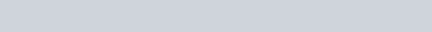# Specific speed

Specific speed is a term used to describe the geometry (shape) of a pump impeller. People responsible for the selection of the proper pump can use this Specific Speed information to :

• Select the shape of the pump curve.
• Determine the efficiency of the pump.
• Predict N.P.S.H. requirements.
• Select the lowest cost pump for their application.

Specific speed is defined as “the speed of an ideal pump geometrically similar to the actual pump, which when running at this speed will raise a unit of volume, in a unit of time through a unit of head”.

The performance of a centrifugal pump is expressed in terms of pump speed, total head, and required flow. This information is available from the pump manufacturer’s published curves. Specific speed is calculated from the following formula, using data from these curves at the pump’s best efficiency point (bep.):N = The speed of the pump in revolutions per minute (rpm.)

Q = The flow rate in liters per second ( for either single or double suction impellers)

H = The total dynamic head in meters

Please refer to the following chart:• Note: dividing the US units by 51.64 will yield the SI units

Pumps are traditionally divided into three types: radial flow, mixed flow, and axial flow. When you look at the above chart you can see there is a gradual change from the radial flow impeller, which develops pressure principally by the action of centrifugal force, to the axial flow impeller, which develops most of its head by the propelling or lifting action of the vanes on the liquid.

In the specific speed range of approximately 1000 to 6000 double suction impeller are used as frequently as the single suction impellers.

If you substitute other units for flow and head the numerical value of Ns will vary. The speed is always given in revolutions per minute (rpm.). Here is how to alter the Specific Speed number (Ns) if you use other units for capacity and head :

• United States ….Q = G.P.M. and H = feet. Divide the Ns by 1.63
• British …………Q = Imp.G.P.M. and H = feet. Divide the Ns by 1.5
• Metric …………Q = M3/hour and H = meters. Divide the Ns by 1.9

As an example we will make a calculation of Ns in both metric and U.S. units :

• Q= 110 L/sec. or 396 M3/ hour or 1744 G.P.M.
• H = 95 meters or 312 feet
• Speed = 1450 rpm.If the above results were describing an actual application, we would notice that it was a low specific speed, radial flow pump, meaning It would be a large pump with a low efficiency. Going to 2900 rpm. or higher would increase the Ns to 1000 or more, meaning a smaller pump with a much higher efficiency, but this higher rpm. would have other possible consequences :

• The higher efficiency would allow you to use a less powerful driver that would reduce your operating costs.
• A smaller pump makes associated hardware cheaper. For instance, a smaller diameter shaft means a lower cost mechanical seal and lower cost bearings.
• Cavitation could become a problem as the increase in speed means an increase in the N.P.S.H. required.
• If you are pumping an abrasive fluid, abrasive wear and erosion will increase with increasing speed.
• Many single mechanical seals have problems passing fugitive emission standards at the higher pump speeds.
• High heat is a major cause of bearing failure. The higher pump speeds contribute to the problem.

The following diagram illustrates the relationship between specific speed and pump efficiency. In general, the efficiency increases as Ns increases.Specific speed also relates to the shape of the individual pump curve as it describes head, capacity, power consumption and efficiency.In the above diagram you will note that :

• The steepness of the head/ capacity curve increases as specific speed increases.
• At low specific speed, power consumption is lowest at shut off and rises as flow increases. This means that the motor could be over loaded at the higher flow rates unless this was considered at the time of purchase.
• At medium specific speed the power curve peaks at approximately the best efficiency point. This is a non overloading feature meaning that the pump can work safely over most of the fluid range with a motor speed to meet the bep. requirement.
• High specific speed pumps have a falling power curve with maximum power occurring at minimum flow. These pumps should never be started with the discharge valve shut. If throttling is required a motor of greater power will be necessary.
• As a rule of thumb, lower specific speeds produce flatter curves, while higher specific speeds prouce steeper ones

Keep in mind that efficiency and power consumption were calculated at the best efficiency point (bep.) In practice most pumps operate in a throttled condition because the pump was oversized at the time it was purchased. Lower specific speed pumps may have lower efficiency at their bep., but at the same time will have lower power consumption at reduced flow than many of the higher specific speed designs.

The result is that it might prove to be more economical to select a lower specific speed design if the pump has to operate over a broad range of capacity.

### Posted

• On February 18, 2018